Éducation par Blue Lightning Labs

# Mathemagics: Mental Math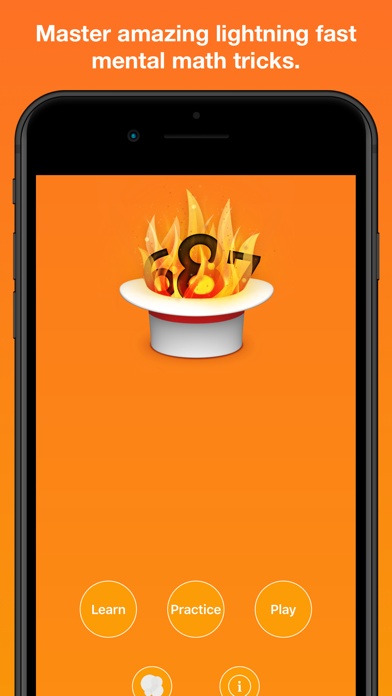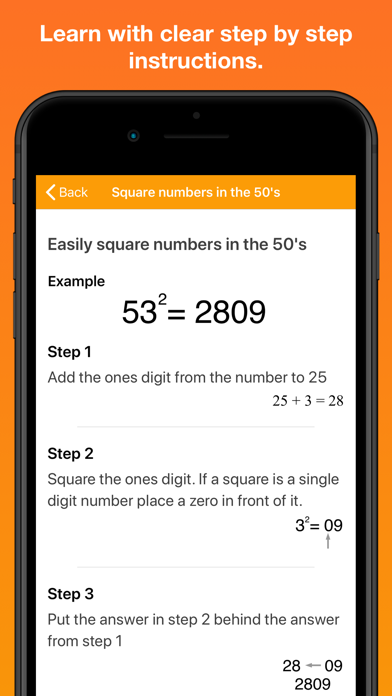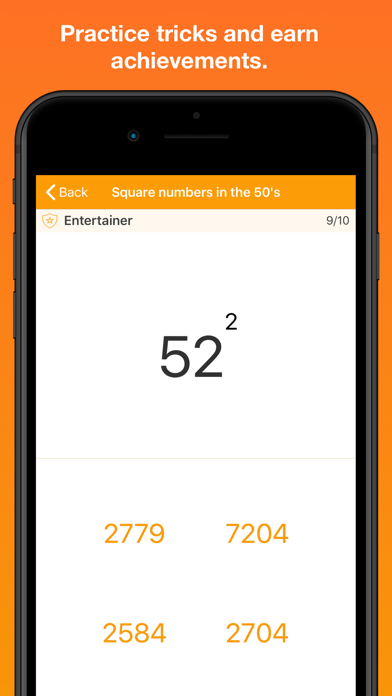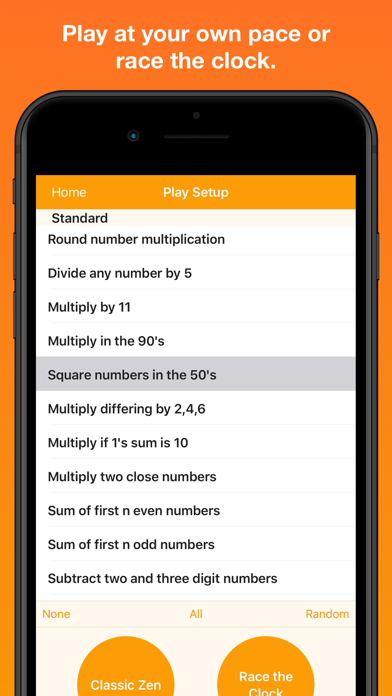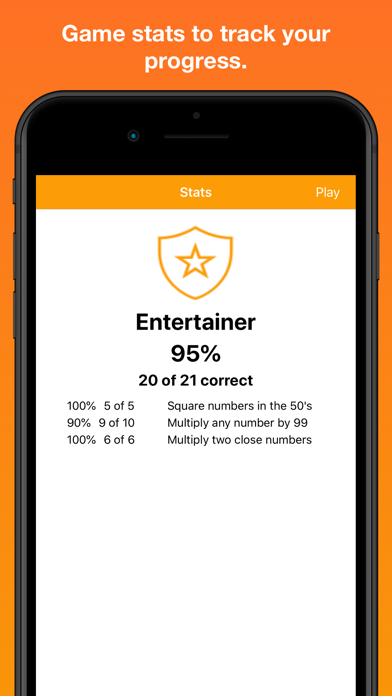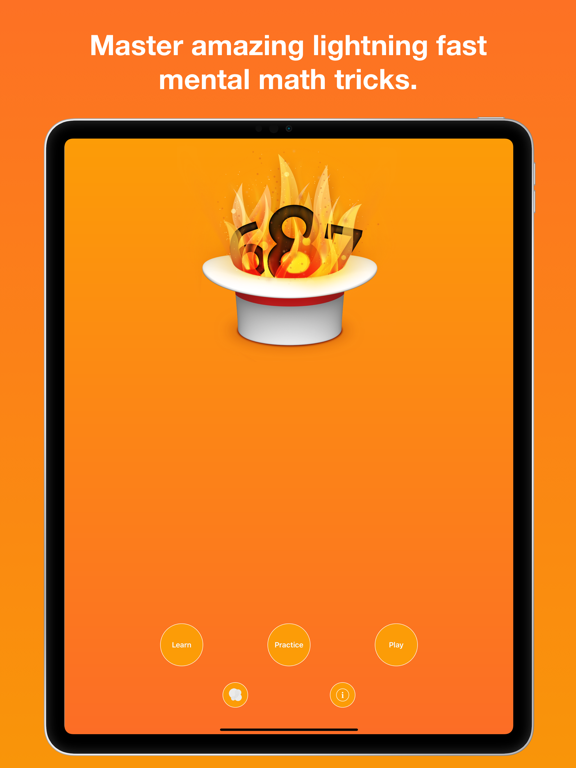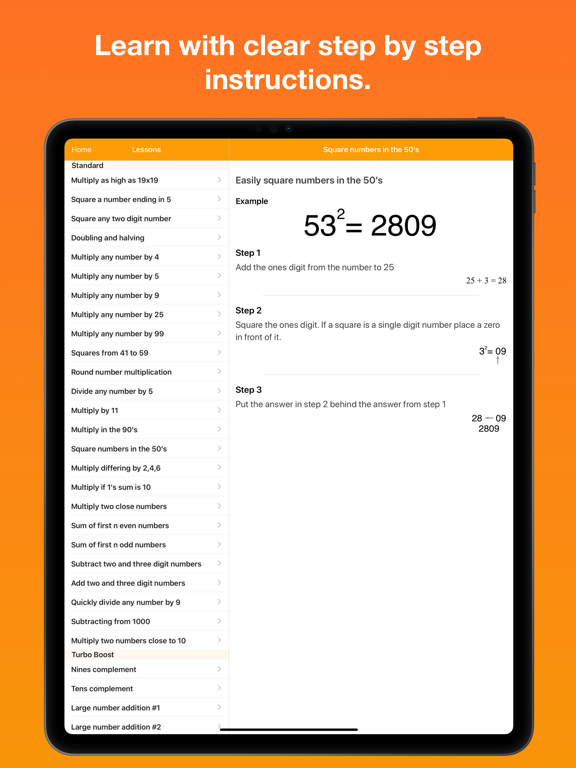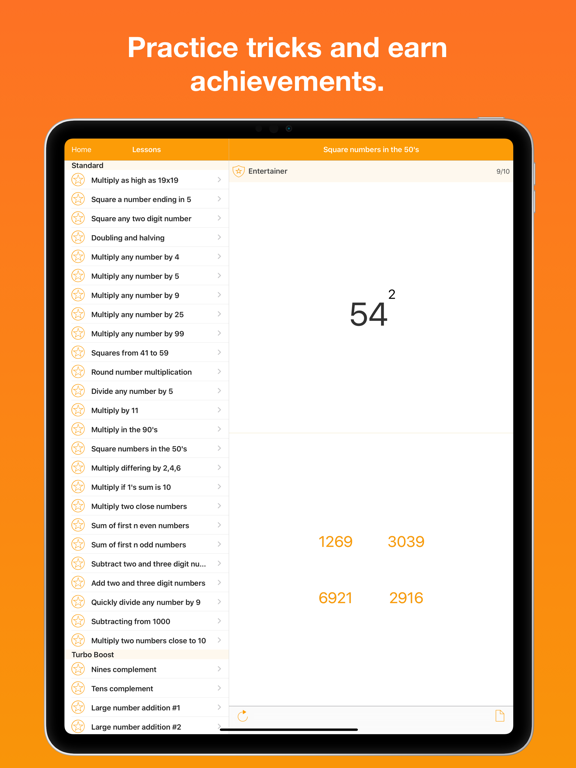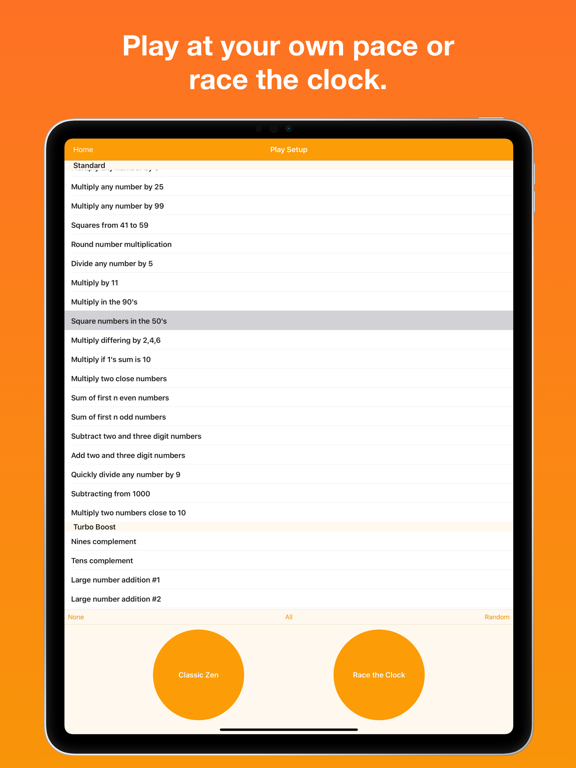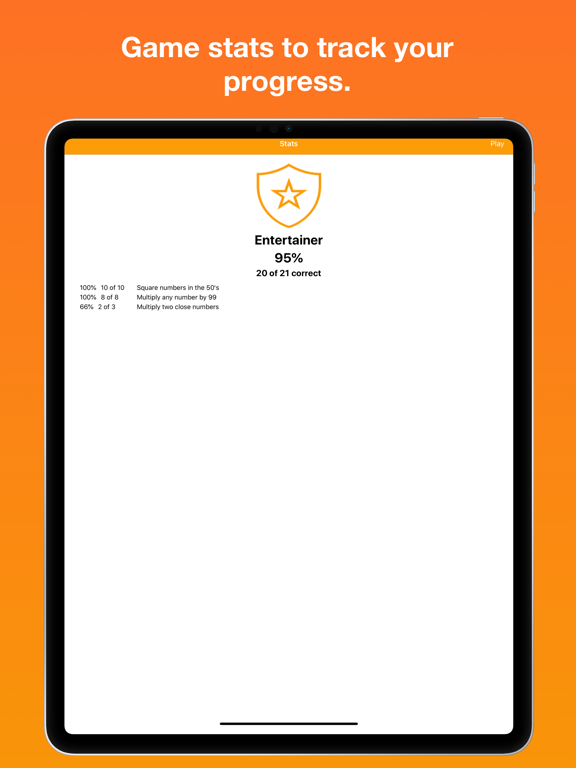⭐⭐⭐⭐✨ (11 votes, moyenne : 4,73 / 5)

Version : 6.3.0

Compatibilité : iOS 12.0 +

Taille : 22,42 Mo

Prix : 5,99 €##### Description
Amaze and delight others as you multiply, divide, and square at lightning fast speed. Learn and practice the tricks of mental math calculation in a fun and engaging application. Study any of the math tricks and then practice them as you progress through various levels of proficiency. Tease your brain with a quick practice session while waiting in lines, riding in the car, or on the plane. Only the basic math skills of addition, subtraction and simple multiplication and division are needed to achieve astonishing results.

Students: Baffle your math teachers by providing answers faster than thought possible.
Teachers: Inspire your students as you pull answers to math problems out of thin air.

Master these math tricks:
* Multiply as high as 20×20
* Square a number ending in 5
* Square any two digit number
* Doubling and halving
* Multiply any number by 4
* Multiply any number by 5
* Multiply any number by 9
* Multiply any number by 25
* Multiply any number by 99
* Squares from 41 to 59
* Round number multiplication
* Divide any number by 5
* Multiply by 11
* Multiply in the 90’s
* Square numbers in the 50’s
* Multiply differing by 2,4,6
* Multiply if 1’s sum is 10
* Multiply two close numbers
* Sum of first n even numbers
* Sum of first n odd numbers
* Subtracting two and three digit numbers
* Adding two and three digit numbers
* Quickly divide any number by 9
* Subtracting from 1000 and higher multiples of 10
* Multiply two numbers close to 10
* Nines complement
* Tens complement
* Large number subtraction
* Evenly divisible by 3
* Evenly divisible by 4
* Evenly divisible by 5
* Evenly divisible by 6
* Evenly divisible by 7
* Evenly divisible by 8
* Evenly divisible by 9
* Evenly divisible by 10
* Evenly divisible by 11
* Evenly divisible by 12
* Square numbers in the 20’s
* Square numbers in the 30’s
* Square numbers in the 60’s
* Square numbers in the 70’s
* Square numbers in the 80’s
* Square numbers in the 100’s
* Square numbers in the 200’s
* Square numbers in the 300’s
* Square numbers in the 400’s
* Square numbers in the 500’s
* Square numbers in the 700’s
* Square numbers in the 800’s
* Square numbers in the 900’s
* Casting Out Nines Digit Sum
* Check Addition by Casting Nines
* Check Subtraction by Casting Nines
* Check Multiplication by Casting Nines
* Check Division by Casting Nines
* Casting Out Elevens Digit Sum
* Check Addition by Casting Elevens
* Check Subtraction by Casting Elevens
* Check Multiplication by Casting Elevens
* Check Division by Casting Elevens

Prepare for the math section on the SAT, PSAT, ACT, GRE, GMAT, MCAT, or any other standardized test. Don’t waste time with a calculator on the few tests that allow them. Use Mathemagics as a tutor to quicken up your test taking abilities.

Perfect training for the UIL Mathematics and Number Sense Contests.

Activate and challenge your brain to expand its ability to think efficiently and have fun at the same time. Train yourself to break problems down in to smaller more manageable pieces in order to reach solutions quicker and more accurately than anyone else.

=================================

“Best New iPhone Education App”
– eduinreview.com

May 2012
Featured in the App Store Test Prep promotion

December 2011
Featured as an AppStore Education Staff Favorite
Featured in the AppStore Hall of Fame

March 16, 2010
Featured in the EU AppStores as New and Noteworthy

December 8, 2009
Featured as an AppStore Best of 2009 App

November 17, 2009
Featured as an AppStore Essential (Apps for Boys)

September 22, 2009
Featured as an AppStore Essential (High School Survival Guide)

August 25, 2009
Featured as an AppStore Staff Favorite

=================================

=================================

Version 6.3.0

##### Changements récents
* Bug fixes
* Compatibility update